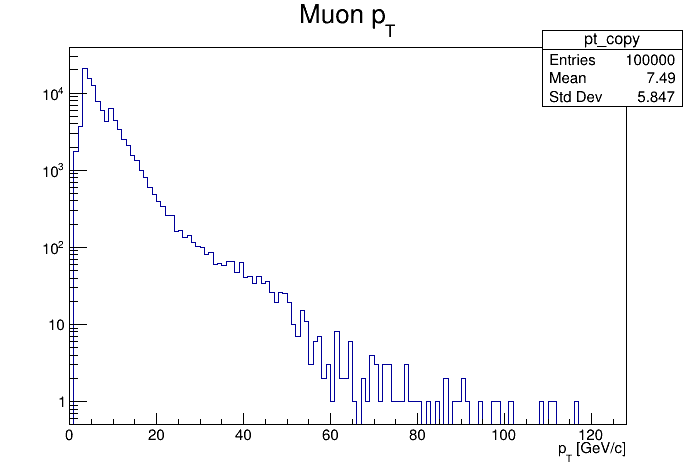# Df 0 1 5_ Lazy Data Source¶

Use the lazy RDataFrame data source to concatenate computation graphs.

This tutorial illustrates how to take advantage of a lazy data source creating a data frame from columns of one or multiple parent dataframe(s), delaying the creation of the columns to the actual usage of the daughter data frame. Dataset Reference: McCauley, T. (2014). Dimuon event information derived from the Run2010B public Mu dataset. CERN Open Data Portal. DOI: 10.7483/OPENDATA.CMS.CB8H.MFFA. From the ROOT website: https://root.cern.ch/files/tutorials/tdf014_CsvDataSource_MuRun2010B.csv

Author: Danilo Piparo (CERN)
This notebook tutorial was automatically generated with ROOTBOOK-izer from the macro found in the ROOT repository on Tuesday, June 15, 2021 at 07:18 AM.

In :
using namespace ROOT::RDF;


Let's first create a rdf that will read from the csv file. See the tutorial relative to CSV data sources for more details!

In :
auto fileNameUrl = "http://root.cern.ch/files/tutorials/df014_CsvDataSource_MuRun2010B.csv";
auto fileName = "df015_CsvDataSource_MuRun2010B.csv";
if(gSystem->AccessPathName(fileName))
TFile::Cp(fileNameUrl, fileName);

auto csv_rdf = MakeCsvDataFrame(fileName);

[TFile::Cp] Total 14.46 MB	|====================| 100.00 % [92.8 MB/s]


Now we take out four columns: px and py of the first muon in the muon pair

In :
std::string px1Name = "px1";
auto px1 = csv_rdf.Take<double>(px1Name);
std::string py1Name = "py1";
auto py1 = csv_rdf.Take<double>(py1Name);


Now we create a new dataframe built on top of the columns above. note that up to now, no event loop has been carried out!

In :
auto df = MakeLazyDataFrame(std::make_pair(px1Name, px1), std::make_pair(py1Name, py1));


We build a histogram of the transverse momentum the muons.

In :
auto ptFormula = [](double px, double py) { return sqrt(px * px + py * py); };
auto pt_h = df.Define("pt", ptFormula, {"px1", "py1"})
.Histo1D<double>({"pt", "Muon p_{T};p_{T} [GeV/c];", 128, 0, 128}, "pt");

auto can = new TCanvas();
can->SetLogy();
pt_h->DrawCopy();

return 0;


Draw all canvases

In :
gROOT->GetListOfCanvases()->Draw()